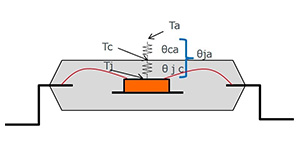# Definitions and Heat Generation

2018.07.26

・Losses are the difference between the input power and the output power, or the reciprocal of the efficiency.

・The junction temperature is the ambient temperature plus heat generation, where heat generation is losses × thermal resistance (θj-a).

・Losses are converted into heat, and therefore are an important subject for study.

Upon undertaking to study losses, we first present definitions relating to losses, and review heat generation and junction temperatures.

## Losses and Efficiency

We begin by reviewing the definition of efficiency and its relation to losses. Efficiency is the fraction of output power relative to input power. This definition arises because, when an input power is converted into a desired output, losses always occur. Hence when losses are expressed as a fraction or ratio, they become the reciprocal of the efficiency, or as a power value, losses are the result of subtracting the output power from the input power. Thus losses can be represented by a number of equations.

Efficiency＝Output power÷Input power [%]
Losses＝1－Efficiency [%]
Losses＝Input power－Output power [W]
Losses＝Output power×(1－Efficiency)÷Efficiency [W]

## Losses and Junction Temperature

Losses are studied and evaluated because losses are converted into heat. That is, when confirming that the junction (chip) temperature, which is the important maximum rating, is within the specified value range, and that the device may be used under the applicable conditions, heat generation is an important quantity to be studied. The junction temperature Tj is expressed as follows.

Tj [℃]＝Ta [℃]＋（θj-a [℃/W]×Losses [W]）

In this equation, the quantity θj-a[°C/W]×Losses [W] has been placed in parentheses; this quantity is the heat generation. In other words, Tj is just the ambient temperature Ta plus the heat generation. Below, the thermal resistance of the package and its definition are given.

The thermal resistance θj-a differs depending on the package and the mounted board conditions. Ordinarily, a standard value is given on the IC data sheet.## Definition

θja Thermal resistance between junction temperature （Tj） and ambient temperature（Ta）
θjc Thermal resistance between junction temperature （Tj） and case surface temperature（Tc）
θca Thermal resistance between case surface temperature（Tc） and ambient temperature (Ta)
Tj Junction temperature
Ta Ambient temperature
Tc Case surface temperature